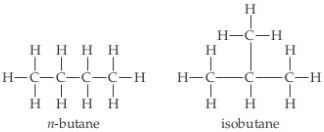# Problem: The structural formulas of the compounds n-butane and isobutane are shown below.Determine the molecular formula of each.

###### FREE Expert Solution

the molecular formula is the unsimplified and actual formula of a compound.###### Problem Details

The structural formulas of the compounds n-butane and isobutane are shown below.Determine the molecular formula of each.